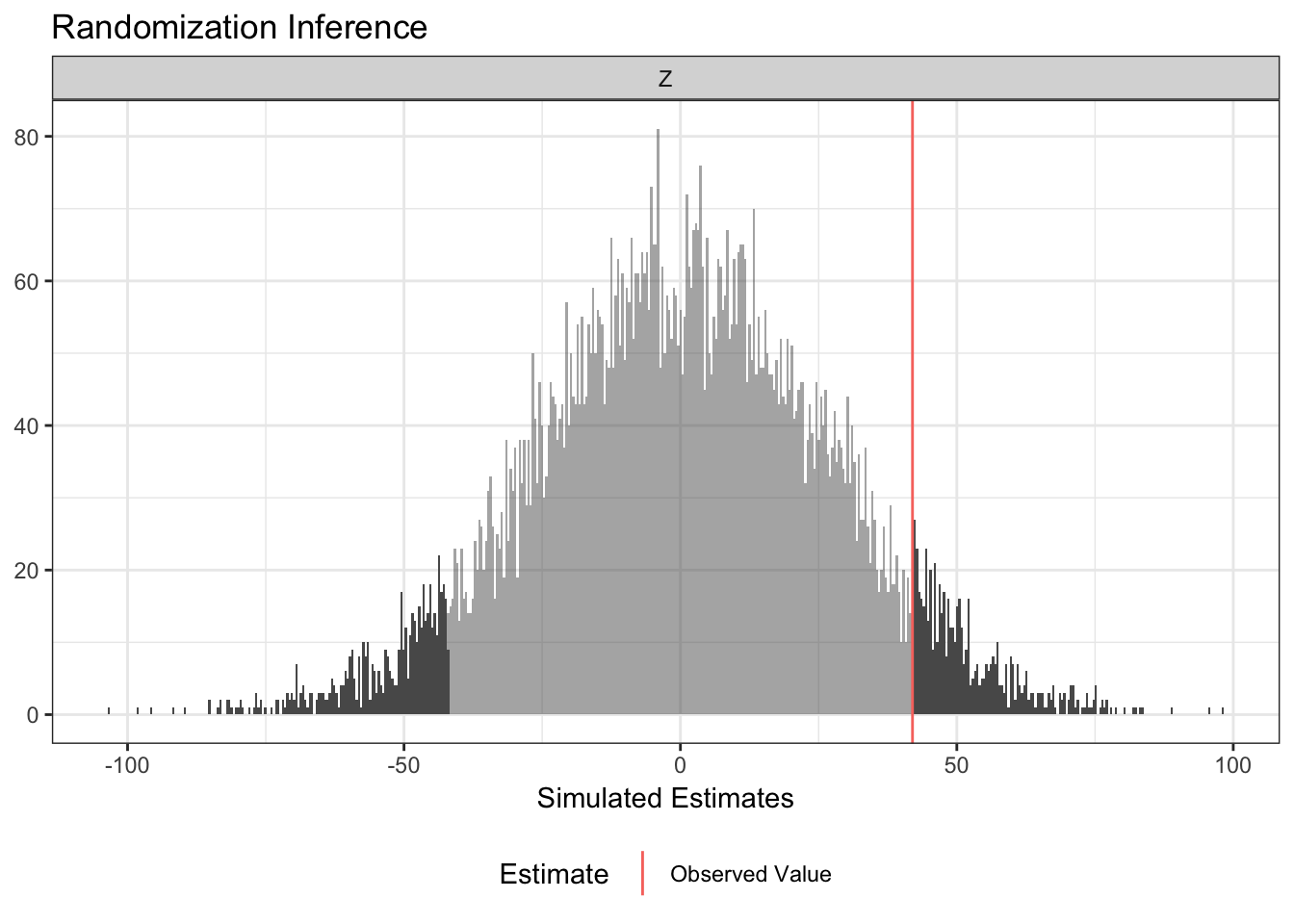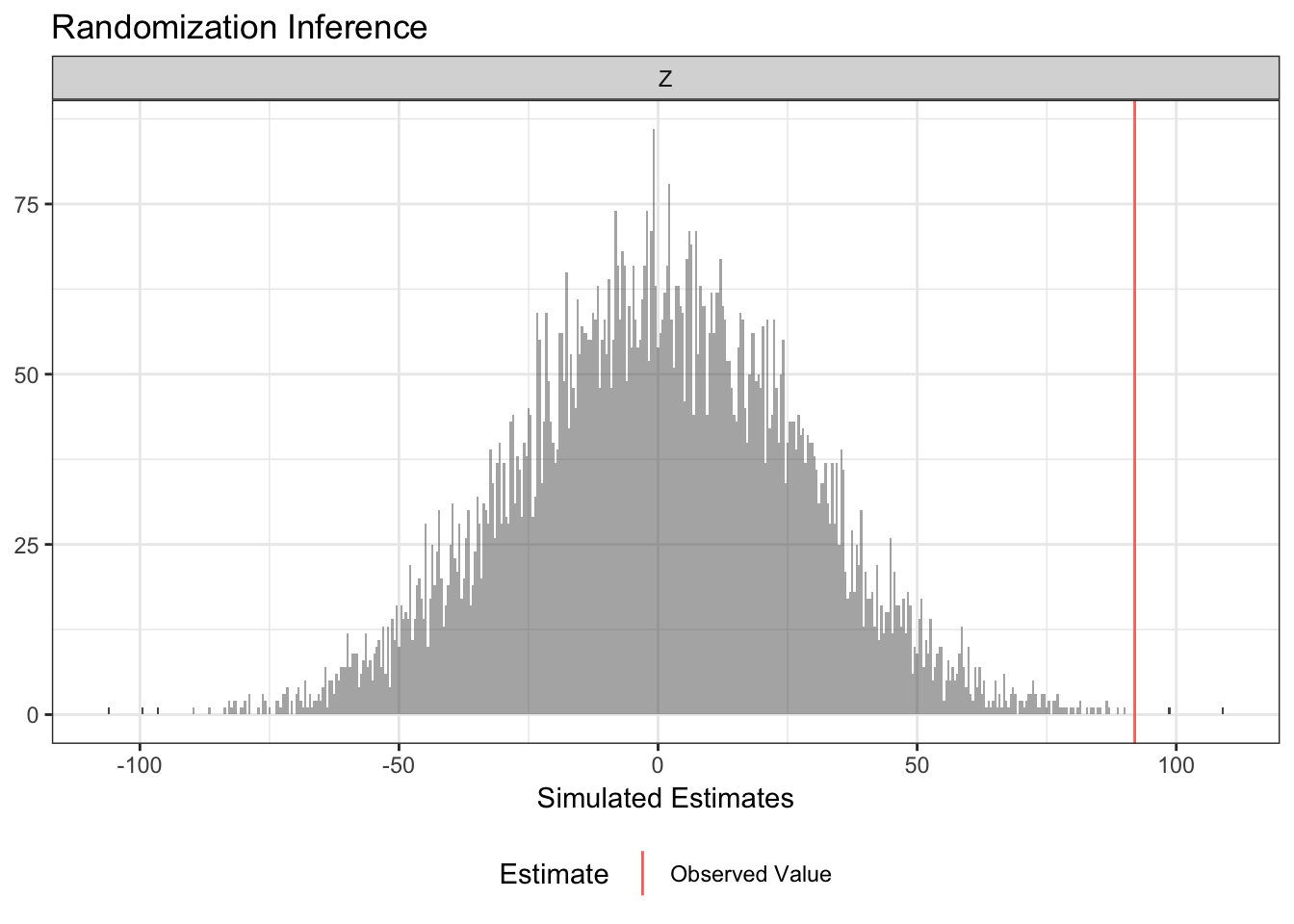Randomization inference is a procedure for conducting hypothesis tests that takes explicit account of a study’s randomization procedure. See 10 things about Randomization Inference for more about the theory behind randomization inference. In this guide, we’ll see how to use the ri2 package for r to conduct 10 different analyses. This package was developed with funding from EGAP’s innaugural round of standards grants, which are aimed at projects designed to improve the quality of experimental research.

To illustrate what you can do with `ri2`, we’ll use some data from a hypothetical experiment involving 200 students in 20 schools. We’ll consider how to do randomization inference using a variety of different designs, including complete random assignment, block random assignment, cluster random assignment, and a multi-arm trial. You can check the kinds of random assignment methods guide for more on the varieties of random assignment.

# 1. Randomization inference for the Average Treatment Effect

We’ll start with the most common randomization inference task: testing an observed average treatment effect estimate against the sharp null hypothesis of no effect for any unit.

In `ri2`, you always “declare” the random assignment procedure so the computer knows how treatments were assigned. In the first design we’ll consider, exactly half of the 200 students were assigned to treatment using complete random assignment.

``````library(ri2)
complete_dec <- declare_ra(N = 200)``````

Now all that remains is a call to `conduct_ri`. The `sharp_hypothesis` argument is set to `0` by default corresponding to the sharp null hypothesis of no effect for any unit. We can see the output using the `summary` and `plot` commands.

``````sims <- 10000

ri_out <-
conduct_ri(
Y ~ Z,
declaration = complete_dec,
sharp_hypothesis = 0,
data = complete_dat,
sims = sims
)

summary(ri_out)``````
``````##   coefficient estimate two_tailed_p_value null_ci_lower null_ci_upper
## 1           Z    41.98             0.1168      -50.9435        51.223``````
``plot(ri_out)``You can obtain one-sided p-values with a call to `summary`:

``summary(ri_out, p = "upper")``
``````##   coefficient estimate upper_p_value null_ci_lower null_ci_upper
## 1           Z    41.98         0.061      -50.9435        51.223``````
``summary(ri_out, p = "lower")``
``````##   coefficient estimate lower_p_value null_ci_lower null_ci_upper
## 1           Z    41.98        0.9391      -50.9435        51.223``````

# 2. Randomization inference for alternative designs

The answer that `ri2` produces depends deeply on the randomization procedure. The next example imagines that the treatment was blocked at the school level.

``````blocked_dat <- read.csv("blocked_dat.csv")
blocked_dec <- declare_ra(blocks = blocked_dat\$schools)

ri_out <-
conduct_ri(
Y ~ Z,
declaration = blocked_dec,
data = blocked_dat,
sims = sims
)
summary(ri_out)``````
``````##   coefficient estimate two_tailed_p_value null_ci_lower null_ci_upper
## 1           Z    91.98              5e-04       -53.821         52.76``````
``plot(ri_out)``A very similar syntax accommodates a cluster randomized trial.

``````clustered_dat <- read.csv("clustered_dat.csv")
clustered_dec <- declare_ra(clusters =  clustered_dat\$schools)

ri_out <-
conduct_ri(
Y ~ Z,
declaration = clustered_dec,
data = clustered_dat,
sims = sims
)
summary(ri_out)``````
``````##   coefficient estimate two_tailed_p_value null_ci_lower null_ci_upper
## 1           Z    79.32             0.0095       -63.242        62.282``````
``plot(ri_out)``